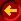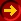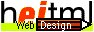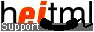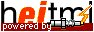# Dynamic Pages

Good Morning,

While surfing the Web you may have found Web pages that change their appearance and contents as time goes by. Newspaper and magazine Web sites change frequently, sometimes daily or even hourly. Often these changes come about when someone puts a new version of a Web page on the Web server. But there are also pages that automatically update themselves, drawing information from database and other resources not part of the original content. We call these pages dynamic pages.

With dynamic pages, Web masters perform fewer administrative tasks to keep their pages current. It makes Web pages more interesting to Web users, and interesting Web pages are more likely to be revisited.

This page is itself a dynamic page. The greeting at the beginning of the page changes depending on the time of the day. Note that the current time of a computer's clock is a resource available to the Web server. Other examples of resources available to the Web server are the IP address of the client that has connected to the server and the identification of the kind of agent (browser) the client is using.

 Technical Note: The process of generating a dynamic page is called interpretation or evaluation, the process itself is called an interpreter. The result of an evaluation is a value which is text (a string) in the case of the dynamic page.

heitml provides some of the resources available to the Web server in the form of server variables (See the Server Variables page in the Language Reference for a complete list). Variables are best thought of as boxes in which information is stored. They have a name, a type and a value. For example, the time is available through a server variable named SrvTime. Its value is a large number which is the sum of hours multiplied by ten million, minutes multiplied by 100,000, seconds multiplied by a thousand, and the milliseconds (e.g. this page was generated at 00:30:25 and 323 milliseconds. Thus, the SrvTime value is 3025323). The data type for the SrvTime value is an integer.

Next we want to output the greeting depending on the value of SrvTime. In the morning, before noon, we like to say "Good morning". In the evening, after 6 p.m., we want to say "Good evening" and in between we simply want to say "Hi".

To achieve this, we must first take a look at the if Tag in its most basic form:

 ``` Do This Do This Do That ```

Conditions can evaluate to either true or false. The data type which defines logical values true or false is called Boolean. If a condition in the if or elsif Tag evaluates to true, the code between the condition and the elsif or else is evaluated. If all conditions evaluate to false, the code between the final else and </if> is evaluated.

Here's how we can use the if tag to greet people accordingly.

 ``` Good Morning Good Evening Hi ```

The first condition SrvTime<120000000 checks whether the current value of SrvTime is less than the value for 12a.m., which is 120000000. The value 120000000 is not a variable, but a constant. A constant differs from a variable in that it has a fixed value and type. The data type of the constant 120000000 is integer. The < sign is called an operator. It operates on two values and is therefore called a binary operator. The value on the left-hand side is called the left operand, the value on the right-hand side is called the right operand. To evaluate the < operator successfully both operands must have the same type. The < operator evaluates to true if the value of the left operand is less than the value of the right operand and it evaluates to false otherwise. Since the < operator can only evaluate to either true or false, it is a Boolean operator.

### Expressions

The actual usage of a constant, variable or operator with its operands is called an expression. The operators that you can use in an expression are most probably known to you from math: + - * / %. Other well-known operators are those for comparing values like == != < <= (equal to, not equal to, less than, less or equal than, respectively). Operator operands may contain expressions called sub-expressions, so expressions have a tree structure with the root at the top. You may want to parenthesize sub-expressions to overrule the pre-defined operator precedence. For example, the expression 2*2+2 evaluates to 6, but 2*(2+2) evaluates to 8.

Like variables and constants, expressions have a type. heitml handles all frequent data types such as Integer(32-Bits), Real(double precision, 64-Bits), Boolean(true or false), String, Tuple, types (classes) derived from the Object type and null for an unknown data type. Most operators expect that their operands have a certain type. For more information, read the section about type checking in the Language Reference.

If you need to know whether or not an expression is (for example) integer this can be done easily using the isinteger() heitml built-in function which delivers a true or false value.

Example:

 input: resulting output: `` `true` `` `true` `` `false` `` `false`

From the previous table you can see that heitml built-in functions (see section heitml Functions in the Language Reference) can be used in expressions and how expressions can be evaluated and put on a page using the heitml ? Tag. To just evaluate expressions and store the result, the user can introduce new variables. Storing expressions in variables is done with the assignment operator =. The type of a variable is handled dynamically which means that a variable need not to be declared with certain type and that its type can change by simply changing the data it contains. Note, that this is not possible with Server Variables.

Variables are all local to either the current heitml-page or user-defined tag with the exception of three global tuple variables gl (global variables), ff (form variables) and se (session variables) which can be accessed everywhere.

We'll start off with a comprehensive example of how variables, in this case Integer variables are put to work to do calculations.

 ``` ```

As you can see here giving your variables obvious names helps a lot in making your code comprehensive and it will work wonders in more lengthy codes. In this case totalprice is just that, the total price. One of the benefits of using variables is obvious in this example. If you want to know the total price of any other product all you need to do is change the price variable and totalprice will give you the price plus tax. Without variables you would be stuck having to calculate again and again for every new price.

One last thing about the names you give your variables. You have complete freedom, except for emptytuple, null, true and false which have been declared by heitml and cannot be used as your own variables. For more detailed information see the section about the Lexical Structure of heitml.

Something very similar can be done using string variables, too. In the next example let's assume you would like to know from which country your guest is. SrvHost will give you your guests address which as an appendix contains all we will need, to know where he's from. This is yet another nice example for a dynamic page.

 ``` Welcome to my Web site! Willkommen auf meiner Web site!> ```

The built-in function right() does all the work for us here. In this example it scans the variable for the dot from right to left and then gives us information about what follows to the right of the dot. If our guest's address ends with a "com" he is from the United States and if it ends with a "de" he is from Germany and both are greeted in their own language.

We will finish this section with a quick and real-life example for a dynamic page. Look at the copyright message at the bottom of this page. There you'll find the year until the copyright is valid. In most cases, this is the ongoing year. To save a Web master from updating a tag definition or even lot of pages at the beginning of each year, you can use an expression with the Server Variable SrvDate which gives you the current date. Like the Server Variable SrvTime which we introduced at the top of this page, SrvDate is an integer variable holding a big number which is the sum of the year multiplied by 10000, the month multiplied by 100 and the day. For example, today is Sunday September 24 2023 and so the value of SrvDate is:

Example:

 input: resulting output: `` `20230924`

Now, the current year can be put on a page by the following expression:

Example:

 input: resulting output: `` `2023`

Again, the ? Tag will evaluate the content of the expression and puts the result on your Web Page. The expression itself consists of the binary division operator / which divides the left operand (in this case a (Server) Variable) by its right operand (a constant). Since, the type of the variable and the constant is integer, the result is also an integer. As you remember from math, the value of the right operand in a division must not be 0.

For more advanced operations on date and time, look at the Date/Time Library.

### Expressions and Modular Pages

Now let's apply what we have learned in the previous section about expressions on what we already know about Modular Pages. Therefore, we take another look at the example that computes the total price for a product.

 ``` ```

Though we only have to replace the price to compute the totalprice for another product, we would still have to copy the three lines everywhere we want to compute a total price. If you still think this is not much work, remember when taxes will increase. Then, you would have to update each page where you copied the code. We will define a tag that will compute the total price and put it in a file totalprice.hei together with a global variable that indicates the tax value.

totalprice.hei
```<let gl.tax = 7>
<def totalprice price>
<return price+price/100*gl.tax>
</def>
```

Our user-defined tag has been enhanced through parameters and expressions. Thus, our tag is really a user-defined function now. Before introducing the concept of user-defined functions, let us complete our example with a Web page template that creates a bill for a product with a special price:

bill.hei
```<HTML>
<include name="mylayout.hei"/>
<include name="totalprice.hei"/>
<TITLE>Bill</TITLE>
<BODY>
<let price = 29.99>
The price is <? price> plus tax (<? gl.tax>%)
Total: <? totalprice(price) "20.2">
Thanks for shopping here!
</BODY>
</HTML>
```

First, our layout and totalprice file is included. Afterwards, some text, the price and the current tax is printed. Our user-defined function totalprice which returns a value of type real can be used in an expression like a built-in function or variable. Though we have no idea of what the total price will be, we know we're only interested in the first two digits behind the dot. The format "20.2" used with the ? Tag will output the value of <total> but only 20 digits in front of and the first two behind the dot. Assuming you're not planning to buy the United States, 20 digits should be enough.

### User-defined Functions

We know there'll be times when you need to define a function for you own purposes. As much as we'd like to provide you with a comprehensive set of Library and Built-in Functions, we can't think of everything, so the next best thing is to allow you to create your own.

Functions are defined as follows:

 Format: ``` ```

The FunctionName is required, of course, but you only have to include as many or as few parameters as needed to complete whatever task you want to perform. Some functions don't require any parameters, but most require one or two. The [heitml] in the above definition means that you can include whatever intermediate statements are necessary to manipulate the passed parameters before arriving at a result. Mostly, however, you'll find that your functions can simply evaluate the parameters in an expression, the result of which is returned or "passed back" to the program.

To illustrate this concept let's first define a couple of simple mathematical functions that require only one parameter. We'll call them sqr and cube because they take a numerical value (real or integer) and raise them to the second and third powers respectively. Here's what they look like:

 input: resulting output: ``` ``` ``` 16 25``` ``` ``` ``` 27 64 64```

As you can see, you can pass parameters to heitml functions any way you like: as pre-defined variables, as constants (sometimes referred to as "immediate" values), or you can even pass another function as an argument (nested functions).

Now we'll go one step further and design a function with two parameters called power, which takes a number x and raises it to the power y:

 input: ``` ``` resulting output: ```81 65536```

There are several interesting things we should point out about the above function. First, you'll notice that we couldn't simply evaluate x and y in a mathematical expression and return the result. We had to perform a series of intermediate steps which included

• initializing a counter variable called i
• preserving the initial value of x by assigning it to variable b
• multiplying b by x
• incrementing the counter variable i
• and repeating the calculation while i < y

We could also have initialized i to a value of 2 and repeated the calculation while i <= y. Either method would have suited our purposes.

Now let's take a look at some functions that manipulate character strings. Here's an example that returns the leftmost "x" number of characters from a string:

 input: `````` resulting output: `1234`

Notice that we used the heitml Built-in Function substring to do most of the work for us.

And now here's a function that returns "x" number of characters starting from the right side of a string:

 input: `````` resulting output: `7890`

OK, by now you should have an idea of what's required to create your own functions, so go ahead and have fun!

### Summary

In this section we introduced the heitml if Tag, variables, expressions and user-defined functions to create Pages that respond dynamically to suit the needs of a given situation, at the same time preserving the structured/modular approach we introduced earlier.

This page was dynamically generated by the web application development tool RADpage of H.E.I. Try the AJAX Article (in German) on www.h-e-i.de.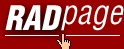Homepage Intro/Features Component Guide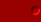Programming Language Guide Modular Pages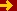Dynamic Pages Browser Data Page Counters Interactive Pages Language Ref. Component Ref. Class Library User Components Tutorial New Features heitml 1User Guide Services Privacy DatenschutzContact / Impressum